# Set Operations : Intersection And Difference Of Two Sets

Intersection and difference of two sets are two different set operations. In set theory, we perform different types of set operations. Such as the intersection of sets, a difference of sets, the complement of sets and the union of sets. It is very easy to differentiate between intersection and union operations. But what is the difference between intersections and the difference of sets? Let us understand here in this article.

## What is an Intersection of Sets?

The intersection of two sets, A and B, which are subsets of the universal set U, is the set which consists of all those elements which are common to both A and B.
It is denoted by the “∩’ symbol. All those elements which belong to both A and B represent the intersection of A and B. Thus we can say that,

A ∩ B = {x : x ∈ A and x ∈ B}

For n sets A1, A2, A3, …, An, where all these sets are the subset of universal set U, the intersection is the set of all the elements which are common to all these n sets.

Depicting this pictorially, the shaded portion in the Venn diagram given below represents the intersection of the two sets A and B.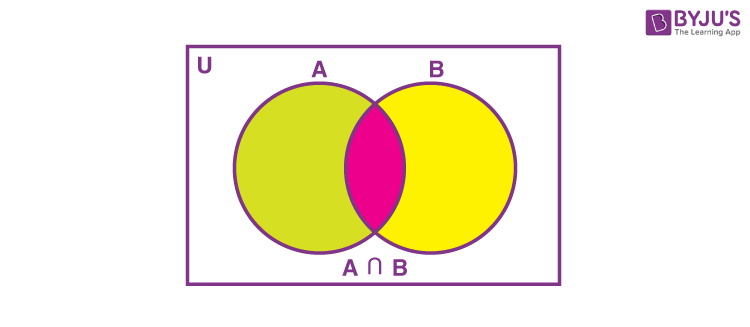Figure 1-Intersection of two sets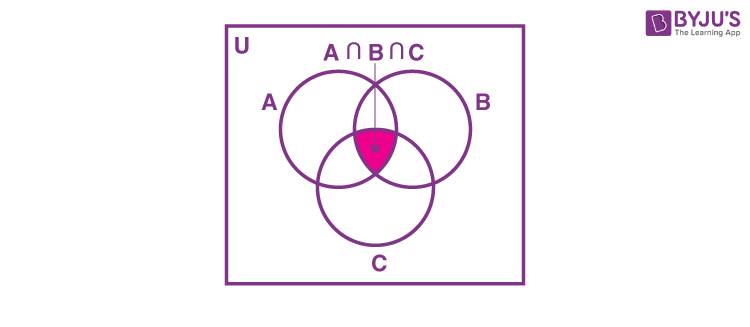Figure 2-Intersection of three sets

## Intersection of Two sets

If A and B are two sets, then the intersection of sets is given by:

$$\begin{array}{l}A \cap B = n(A) + n (B) – n (A \cup B)\end{array}$$

where n(A) is the cardinal number of set A,
n(B) is the cardinal number of set B,
n(AUB) is the cardinal number of the union of sets A and B.

To understand this concept of intersection, let us take an example.

## Example of Intersection of sets

Example: Let U be the universal set consisting of all the n – sided regular polygons where 5 ≤ n ≤ 9. If set A, B and C are defined as:

A = {pentagon, hexagon, octagon}

B = {hexagon, nonagon, heptagon}

C = {nonagon}

Find the intersection of the sets:

i) A and B

ii) A and C

Solution: U = {pentagon , hexagon , heptagon , octagon , nonagon}

i) The intersection is given by all the elements which are common to A and B.

A ∩ B = {hexagon}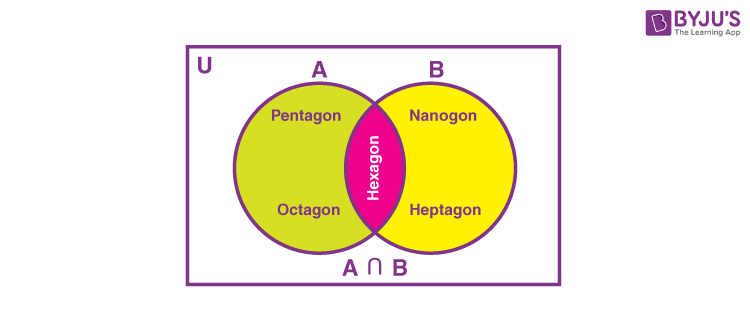ii)  No element is common in A and C. Therefore A ∩ C = ∅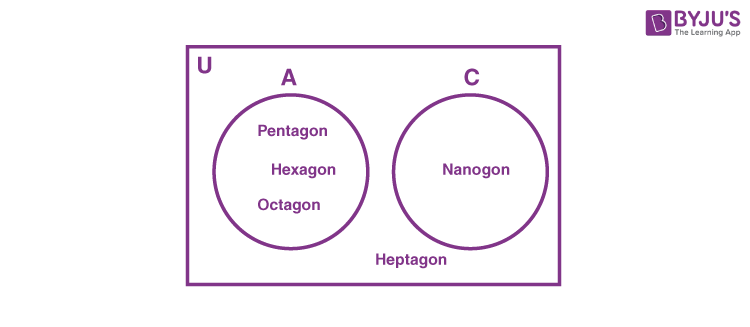Note: If we have two sets X and Y such that their intersection gives an empty set ∅ i.e. X ∩ Y = ∅ then these sets X and Y are called disjoint sets.

## Properties of Intersection of a Set

• Commutative Law: The intersection of two sets A and B follow the commutative law, i.e., A ∩ B = B ∩ A
• Associative Law: The intersection operation follows the associative law, i.e., If we have three sets A , B and C then (A ∩ B) ∩ C = A ∩ (B ∩ C)
• Identity Law: The intersection of an empty set with any set A gives the empty set itself i.e., A ∩ ∅ = ∅
• Idempotent Law: The intersection of any set A with itself gives the set A i.e., A ∩ A = A
• Law of U: The intersection of a universal set U with its subset A gives the set A itself. A ∩ U = A
• Distributive Law: According to this law: A ∩ (B ∪ C) = ( A ∩ B ) ∪ (A ∩ C)

## What is the Difference of Sets?

The difference of the two sets A and B is the set of elements which are present in A but not in B. It is denoted as A-B. In the following diagram, the region shaded in green represents the difference of sets A and B (A-B). And the region shaded in yellow represents the difference of B and A (B-A).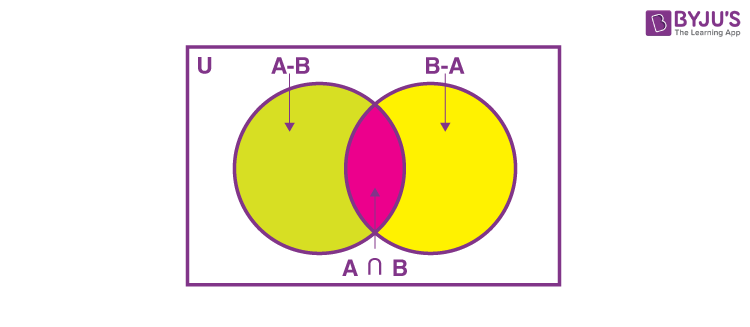## Example of Difference of sets

Let A = {3 , 4 , 8 , 9 , 11 , 12 } and B = {1 , 2 , 3 , 4 , 5 }. Find A – B and B – A.

Solution: We can say that A – B = { 8, 9, 11, 12} as these elements belong to A but not to B

B – A ={1, 2, 5} as these elements belong to B but not to A.

## Video Lesson on What are SetsIt is interesting, isn’t it? There is yet a lot more to explore in Set theory. We here at BYJU’S are paving the way for your learning.

Test your knowledge on Operation On Sets Intersection Of Sets And Difference Of Two Sets